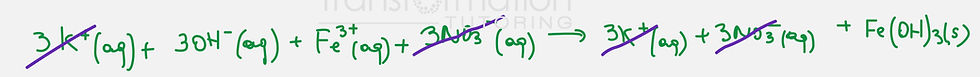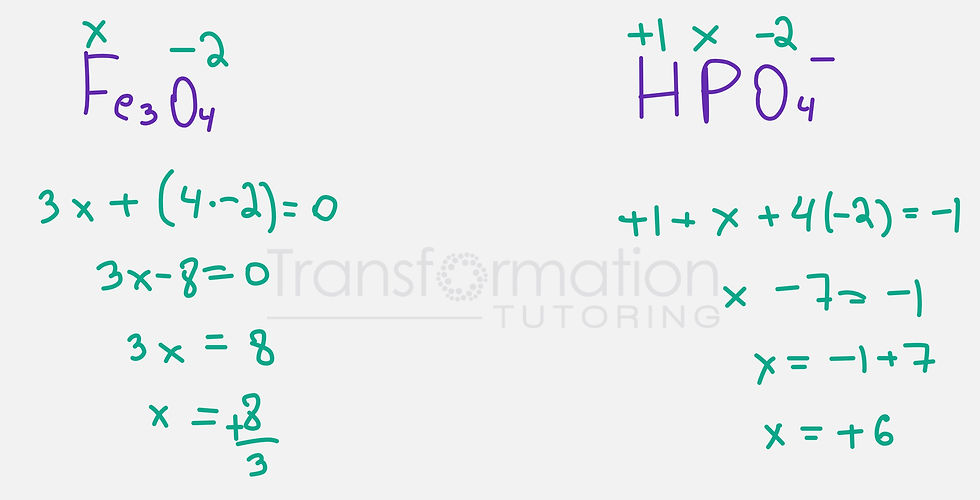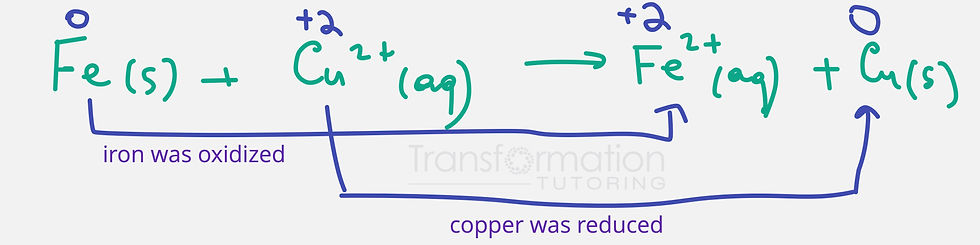top of page
Search

# Chapter 4: Complete Guide to Chemical Reactions (Precipitation, Redox, Neutralization)

Water, the Common Solvent

Water, H2O, is one of the most substances on earth. The intermolecular forces that exist between water molecules are called hydrogen bonds. Many substances can dissolve in water to form aqueous solutions.

Strong and Weak Electrolytes

A solution is a homogeneous mixture (uniform composition).

Solute is a substance that gets dissolved(such as salt).

Solvent is the substance in which the solute dissolves (such as water).

Together, solute and solvent make solution.

Solutions that can conduct electricity are called electrolytes. Solutions that can not conduct electricity are called non electrolytes.

Strong electrolytes are:

• Ionic compounds dissolved in water (metal +nonmetal, metal +polyatomic ion, 2 polyatomic ions, polyatomic ion +nonmetal).

• Strong acids: HCl, HNO3,H2SO4,HBr, HI, HClO4

• Strong bases: Group I or II metals with OH- (Ex: NaOH, KOH)

Weak electrolytes: weak acids and weak bases (Ex: CH3COOH, NH3...)

If the substance is not a strong or weak electrolyte it will be a non electrolyte. Example: C12H22O11

The Composition of Solutions

Solute: substance that dissolves

Solvent: what the solute dissolves in

Solution: solute+solvent

Molarity is used to measure the concentration of a solution

Molarity = M = moles of solute

liters of solution

Molarity problem:

A sample of NaNO3 weighing 0.38 g is placed in a 50.0 mL volumetric flask. What is the molarity of the resulting solution?

First we need to convert grams of NaNO3 into moles using molar mass (found from the Periodic Table).

Molar mass of NaNO3 = 22.99+ 14.007+(3*15.999) = 84.9947 g/mol

0.38 g (1mol/84.9947 g) = 4.47 * 10^-3 mol NaNO3

We also need to convert ml to L by dividing by 1000.

50.0 mL = 0.05L

Now, we can plug everything in

M = 4.47 * 10^-3 mol/0.05L = 0.089 M NaNO3

Dilution

During dilution, water is added to the solution to reach the desired concentration. The formula that can be used for dilution is

MiVi = MfVf

where Mi = initial molarity, Vi =Initial Volume, Mf = Final Molarity, Vf = Final Volume

Problem: You are given a solution of 14.8 M NH3. How many milliliters of this solution do you require to give 100.0 mL of 1.00 M NH3 when diluted

Mi =14.8 M , Vi =? Mf = 100.0 mL Vf = 1.00 M

Now we plug everything in to get:

14.8M(Vi)= (1.00M)(100.0ml) Vi = 6.76 mL

Precipitation Reactions

In a precipitation reaction, two solutions are mixed to produce an insoluble substance. The insoluble substance is called precipitate. We can predict the products of the precipitation reaction the following way:

AB + CD --> AD +CB

Let's go over a step by step guide of how to predict the products and precipitate of a reaction with an example.

Na2SO4(aq) and Pb(NO3)2 --> ?

1. Predict the products by using AB + CD --> AD +CBWe combined K+ with NO3- and Fe^3+ with OH- to predict the products. We also crossed the oxidation numbers to get the correct formulas.

2. Use the solubility table to find the precipitate (insoluble in water). Put (s) next to the precipitate signifying solid. If there is no precipitate, put no reaction in the products.

Rules for Solubility

1. Salts with Group 1 ions are soluble ( Ex: Na+, K+...)

2. Salts with ammonium (NH4+) are soluble

3. Salts with NO3 - are soluble

4. Salts with Cl-, Br- or I- are soluble unless combined with Ag+, Pb^2+ or Hg2^2+

5. Salts with OH- are insoluble unless combined with Group1 metals or NH4+

6. Most sulfide (S^2-), carbonate (CO3^2-), and phosphate (PO4^2-) salts are insoluble

7. Salts with SO4 ^2- are soluble unless combined with Ag+, Ca^2+, Sr^2+, Ba^2+ or Pb^2+KNO3 has K+ which is Group 1 ion and is soluble. Fe(OH)3 is insoluble and that is why we put (s) next to it.

3.Next, we need to balance our equation to make sure we have an equal number of atoms of each element on both sides.4.To write the complete ionic equation, we break all the aqueous ionic compounds into ions. We keep the solid precipitate intact without breaking it.5.The ions that we see on both side are spectator ions. To get the net ionic equation, we can cross them out and write what is left.K+ and NO3- are spectator ions because we see them on both sides of the equationMayya's Trick

To predict the net ionic equation, we put the solid (precipitate) on the right side of the equation and aqueous ions it is made from on the left side of the equation.

Acid-Base ReactionsAccording to Arrhenius definition, acid produces H+ (hydrogen ions or protons) when dissolved in water and base produces OH- (hydroxide ions) when dissolved in water. Examples of acids are HCl, HNO3. An example of base is NaOH.

A strong acid, breaks down(ionizes) in water completely. Similarly, strong bases ionize in water completely. Strong acids and strong bases are strong electrolytes.

Common strong acids are: HCl, HI, HNO3, HBr, H2SO4, HClO4

Commons strong bases contain a group 1 or 2 metal with an OH such as : KOH, NaOH, LiOH, Ba(OH)2

Weak acids and bases ionize into ions partially in water. Weak acids and bases are considered weak electrolytes.

A reaction between an acid and a base is called neutralization reaction. The products on a neutralization reaction are water and salt (ionic compound). To predict the products we simple do AB + CD --> AD +CB like we did before.Net ionic equation for the reaction between strong acid and strong base is:Acid–Base Reactions with Gas Formation

Certain salts react with acids to form gases.

Carbonates react with acids to form CO2 gas

Sulfites react with acids to form SO2 gas

Sulfides react with acids to form H2S gas

Oxidation–Reduction(Redox) Reactions

Another type of reactions is oxidation-reduction reactions which involve a transfer of electrons.

Rules for calculating oxidation numbers

1. Atoms of elements by themselves have an oxidation number of zero. Ex: O2, H2, Fe

2. Ions of elements have an oxidation number equal to the charge. Ex: Cl- has an oxidation number of -1.

3. Oxygen has an oxidation number of -2. Exception: O in H2O2 has an oxidation number of -1.

4. Hydrogen has an oxidation number of +1. Exception: When hydrogen is paired with a metal, its oxidation number will be -1. Example: LiH

5. Group 17 elements (halogens) usually have an oxidation number of -1.

6. Group 16 elements usually have an oxidation number of -2.

7. Group 1 elements usually have an oxidation number of +1.

8. Group 2 elements usually have an oxidation number of +2.

9. The sum of oxidation numbers in a neutral compound is equal to 0.

10. The sum of oxidation numbers in an ion is equal to the charge of the ion.

Let's learn to assign oxidation numbersDescribing Oxidation–Reduction Reactions

Let's learn some special terms for oxidation-reduction reactions.

The reaction can be broken into two halves which are called half reactions. Oxidation is the half reaction where electrons are lost. Reduction is the half reaction where electrons are gained.We can assign oxidation numbers to determine what was oxidized and what was reduced.In the example above, iron went from an oxidation number of 0 to +2, which means it lost two electrons (remember electrons are negative). Losing electrons means oxidation. Copper, on the other hand, went from +2 to 0 by gaining two electrons (reduction).

The molecule where an element got reduced is called an oxidizing agent. A molecule where an element was oxidized is called a reducing agent.

Learn Chemistry with our Online Chemistry Tutor to ace your general chemistry class!